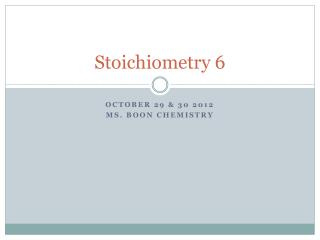DownloadDownload PresentationStoichiometry 6

# Stoichiometry 6

Télécharger la présentation## Stoichiometry 6

- - - - - - - - - - - - - - - - - - - - - - - - - - - E N D - - - - - - - - - - - - - - - - - - - - - - - - - - -
##### Presentation Transcript

1. Stoichiometry6 October 29& 302012 Ms. Boon Chemistry

2. Catalyst: • Today is the third and last day of our copper and silver nitrate stoichiometry lab. • If you could do this lab again, or another one like it, what would you add to the lab to make it more interesting, informative, or fun? Objective: I can analyze laboratory data, identify sources of error, and write a well-reasoned lab report. Agenda: Catalyst Lab Day 3 Exit Slip Review Let’s write a lab report!

3. Warm Up – Lab Day 3 Before we measure the mass of our silver. Let’s watch a video. Focus Question: Describe what you see using scientific vocabulary. (chemical reaction, single-displacement, reactants, products) http://www.youtube.com/watch?v=niy1-u3oI5E

4. Agenda • Catalyst • Lab Day 3 – Data Collection & Begin Calculations • Exit Slip Review • Let’s write a lab report!

5. Lab Day 3: Data Collection (7 minutes) The last step in the lab procedure is to measure the mass of the filter paper with the silver. Each lab group must assign one person to pick up their materials and another person to read the triple beam balance. Record your results in the Day 2/3 data table Begin the Day 3 Calculations while we wait for all groups to finish.

6. Exit Slip 10.25&26 – Standard 3a, 3d, 3e, 3f Balance thechemicalreactionequation. ___Li +__O2 ___Li2O • What is the molar mass of Li? • 7 g/mol • What is the molar mass of Li2O? • 7+7+16 = 30 g/mol • Whatisthe molar mass of O2? • 16 + 16 = 32 g/mol • What is the mole ratio of Li2O to Li in the balanced equation? • 2 mol Li2O • 4 mol Li • What is the mole ratio of Li2O to O2 in the balanced equation? • 2 mol Li2O • 1mol O2 • How many grams Li2O will be produced from 56 g Li? • How many grams Li2O will be produced from 32 g O2? • Identify the limiting reactant and the theoretical (calculated) yield of silver Li2O in grams, when 56 g Li reacts with 32 g O2. • If the actual yield is 30 g Li2O, whatisthepercentyield?

7. Exit Slip 10.25&26 – 3a, 3d, 3e, 3f Review 6. How many grams Li2O will be produced from 56 g Li? 7. How many grams Li2O will be produced from 32 g O2? 8. Identify the limiting reactant and the theoretical (calculated) yield of silver Li2O in grams, when 56 g Li reacts with 32 g O2. 9. If the actual yield is 30 g Li2O, whatisthepercentyield?

8. Let’s Write a Lab Report • Lab Report Criteria: Overview • Length: 4 paragraphs + attach all data, calculations, and observations. • Time: We will work on this today in class. Due today. • Academic standards assessed: • IE 1b – identifying sources of error • W 1.3 – writing a logical and reasoned explanation • Format Requirements: • Title Lab Report: Stoichiometry Lab - Copper and Silver Nitrate Reaction • Introduction Paragraph explains the concept behind the lab. • Procedure Paragraph explains the lab procedure. • Data Analysis Paragraph explains the data and calculations. • Conclusion Paragraph analyzes sources of error and presents next steps.

9. Let’s Write a Lab Report • Lab Report Criteria: Overview • Title Lab Report: Stoichiometry Lab - Copper and Silver Nitrate Reaction • Introduction Paragraph explains the concept behind the lab. • The first sentence should state the purpose of the lab. • Define terms (limiting reactant, excess reactant, theoretical yield; actual yield; percent yield) • Write a descriptive explanation of the single replacement reaction between copper and silver nitrate.

10. Let’s Write a Lab Report • Lab Report Criteria: Overview • Procedure Paragraph explains the lab procedure. • Write at least 5 sentences explaining the specific steps of the lab. (How did you set up the experiment? What did you measure? What did you do on each day? Did you do anything different than the written procedure?)

11. Let’s Write a Lab Report • Lab Report Criteria: Overview • Data Analysis Paragraph explains the data and calculations • What was your theoretical yield and what measurement did you use to calculate it? • What was your actual yield and what measurements did you use to calculate it? • What was your percent yield and what measurements did you use to calculate it? • Attach all calculations.

12. Let’s Write a Lab Report • Lab Report Criteria: Overview • Conclusion Paragraph analyzes sources of error and presents next steps. • Provide at least three sources of error that may have affected your measurements. • Why was your actual yield different than your theoretical yield? • If you were to do a lab like this again, what next steps would you add?